This document is archived and information here might be outdated.  Recommended version.

Geodetic curve support in the ArcObjects Geometry API (ArcObjects .NET 10.8 SDK)
 ArcObjects Help for .NET developers > ArcObjects Help for .NET developers > Developing with ArcGIS > Learning ArcObjects > Managing data > Working with geometry > Creating geometries > Geodetic curve support in the ArcObjects Geometry API

# Geodetic curve support in the ArcObjects Geometry API

Summary
The ArcObjects Geometry application programming interface (API) supports the construction of densified approximations of geodetic curves. In addition, circles and ellipses defined using geodesic distance can be constructed, and the distances between points can be computed using several kinds of geodetic techniques.

### In this topic

A geodetic curve is a one-dimensional curve defined on the surface of a spheroid or sphere, rather than on a plane. The various geodetic curves are defined as follows:
• Geodesic curve - The curve defining the shortest distance between two points on a spheroid.
• Loxodrome (rhumb line) - The curve of constant azimuth. The Mercator projection has the property that a straight line is a loxodrome.
• Great elliptic curve - The curve defined by the intersection of a spheroid surface and a plane passing through the center of the spheroid.
• Normal section curve - The curve defined by the intersection of a spheroid surface and a plane that is normal to the spheroid surface at a specified point.
Polylines and polygons defined using geodetic curves (especially geodesic curves) more accurately represent length, direction, and position everywhere on the surface of the earth. This is something that polylines and polygons that are defined using planar curves cannot do.
A geodesic circle is the locus of points that are a fixed geodesic distance away from a single point. A geodesic ellipse is the locus of points whose geodesic distances from a center point satisfy the ellipse constraint. One way of specifying this constraint is (M*cos(a))^2 + (m*sin(a))^2=D^2, where M is the major axis length, m is the minor axis length, and a is the angle on a reference circle. See the Code sample section for more details.

### Common behavior

A geodetic polyline or polygon (generically referred to as a polycurve) is constructed by first creating it using IConstructGeodetic, then invoking a construction method on it. You can also take an existing polycurve and densify the curve by using IPolycurveGeodetic. A spatial reference (projected or geographic) must either already be associated with the polycurve or be specified as part of the construction. A spatial reference can be obtained from one of the input point parameters of the construction method. If one is present, it will be assigned to the polycurve. If no input point has a spatial reference, then the existing spatial reference of the polycurve is used. If it doesn't have one, the construction cannot be applied and the method returns an error. If the polycurve being constructed is associated with a projected coordinate system, it will be constructed in the corresponding geographic coordinate system (GCS) and projected into the projected coordinate system.
For the line constructors and densification method, vertex attributes are preserved on input vertices, and created vertices have attributes interpolated for them based on length along the geodetic curve. For circle and ellipse constructors, the input center point can have a z value, which will be assigned to all vertices on the boundary of the figure.
For the line constructors and polycurve densification method, the following types of geodetic curves (esriGeodeticType) can be defined:
• esriGeodeticTypeGeodesic
• esriGeodeticTypeLoxodrome
• esriGeodeticTypeGreatElliptic
• esriGeodeticTypeNormalSection
All methods accept a parameter that describes the linear units of input lengths and distances or output lengths. If the unit parameter is not specified, meters are assumed. Angular parameters are specified in the angular units of the GCS associated with the polycurve being constructed. Typically, these will be degrees, but GCSs with other angular units can be defined.
Densification can be controlled through the following methods (esriCurveDensifyMethod):
• esriCurveDensifyByLength - The densification parameter is the curve length increment. Output line segments will be no longer than this value. This method cannot be used when constructing geodesic circles or ellipses.
• esriCurveDensifyByAngle - The densification parameter is an angle increment. Line segments will become shorter as the curvature increases. For geodesic ellipses, the angle is with respect to the reference circle.
• esriCurveDensifyByDeviation - The densification parameter is the deviation from true curve. Line segments of varying length will be generated. The curve parameter specifies the maximum allowable distance between a line segment and the portion of the curve that it is approximating.
The recommended method is esriCurveDensifyByDeviation because it lets you control the absolute ground error of the densified approximation in the most direct and efficient way. The esriCurveDensifyByLength method cannot currently be used with the circle and ellipse constructors.
Geodetic curves that cross the antimeridian will be "unwrapped" to one side of the geographic coordinate system. That is, the longitude sequence (-178, -179, 179, 178) will change to either (-178, -179, -180, -181) or (181, 180, 179, 178).
Geodetic circles and ellipses that cover one or both of the poles are sometimes difficult to represent in a two-dimensional (2D) map. To properly maintain the three-dimensional (3D) polygon in the 2D representation, the polygons covering poles will have pole connector sequences added to them so that they form correct polygons.

### Curve types

In the following image, the Plate-CarrÃ©e projection is used to demonstrate the path of a geodesic curve connecting New York to Berlin. The Great Elliptic and the Normal section curves look similar when displayed on a map, but the geodesic is the most accurate representation of the shortest distance on a spheroid.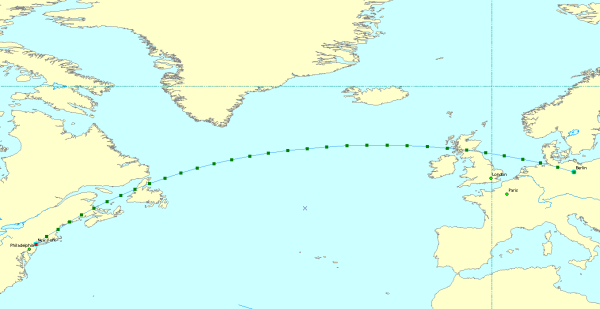In the following image, the loxodrome (rhumb line) curve defines the path taken when starting at New York and travelling 1500 miles along a constant azimuth of 50 degrees. When following a constant azimuth, the loxodrome does not represent the shortest distance on a spheroid, but does make navigation easier.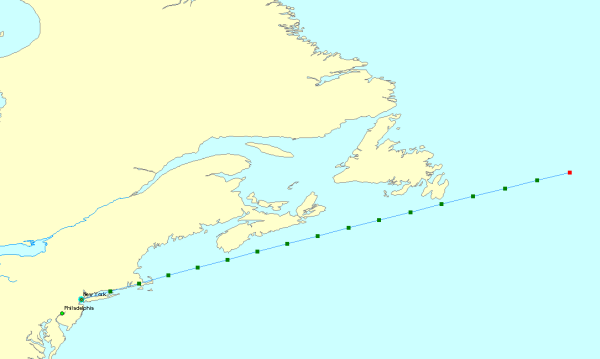### Geodetic curve construction

The Plate-CarrÃ©e projection image described previously demonstrates the construction method by which the only inputs are the endpoints. In the loxodrome image, the inputs for the construction method are the start point, a distance, and an azimuth. The following image is of a geodesic circle that is constructed by choosing a center point and a distance from which all points on the perimeter should lie. In this case, the distance is 500 miles from Kabul as measured on the WGS84 spheroid. The 2D representation of the shape is not circular because it is displayed in the pseudo-Plate-CarrÃ©e projection.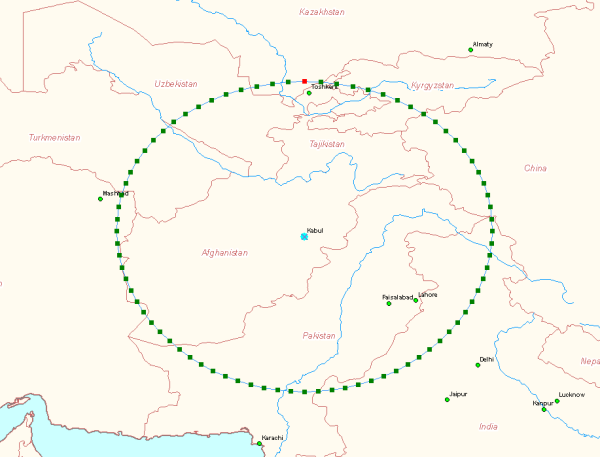A geodesic ellipse is defined by a center, major and minor axes, and an orientation. In the following image, the ellipse is centered on Kabul, has a major axis 800 miles in length, a minor axis 500 miles in length, and is oriented 45 degrees east of north. The straight lines show direction to true north and the 45 degree orientation.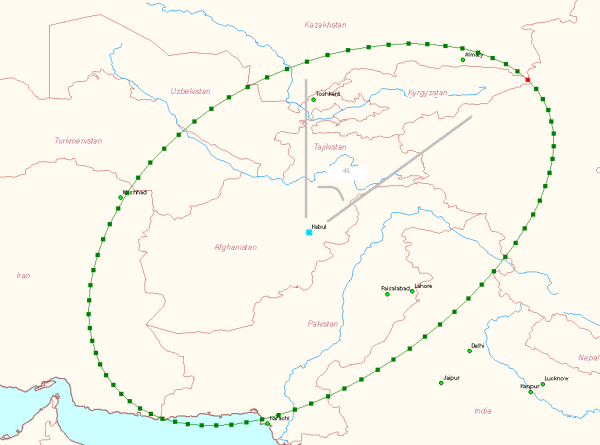### Special cases

This section describes how special cases are handled when constructing geodetic curves and geodetic circles and ellipses.

#### Longitude wrapping

When connecting a pair of points with a geodetic curve, or when densifying a line segment, the shortest way around the spheroid is always chosen. The densified approximation to a geodetic curve will not change the numeric sign of its coordinates when it crosses the antimeridian (the meridian, or longitude line, 180 degrees from the center, or the starting longitude value).
In the following image, a polyline is not densified and is made up of only one line segment (two vertices). The line segment stretches across the prime meridian.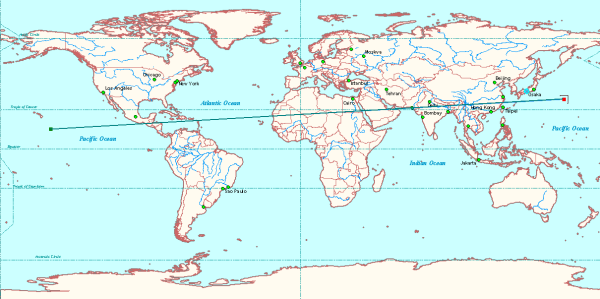When the polyline is densified geodetically, the shortest geodetic line that connects the two endpoints is the path used for the line. The following image shows the densified version of the line: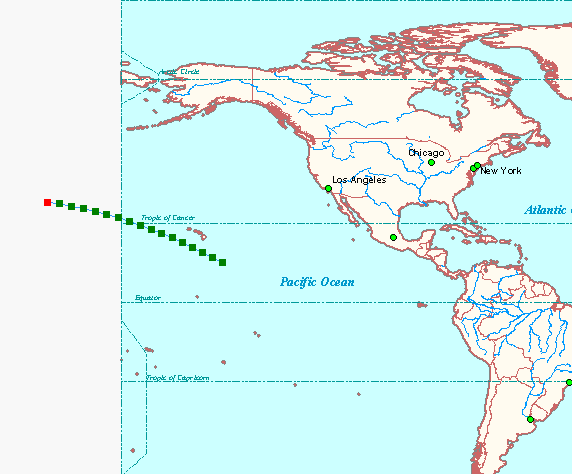#### Pole crossing

A single geodesic curve that passes through a pole is represented as a pair of vertical line segments, plus a pair of "pole connecting segments" to maintain it as a single curve. In the following image, a geodesic curve crosses the North Pole when travelling from New York to Siberia. The pole connecting segments are displayed in the projection as running along the top of the map.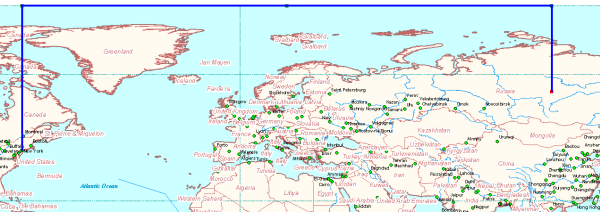In the following image, the same curve is in the North Pole azimuthal equidistant projection. The pair of pole connecting segments project to a point in this projection.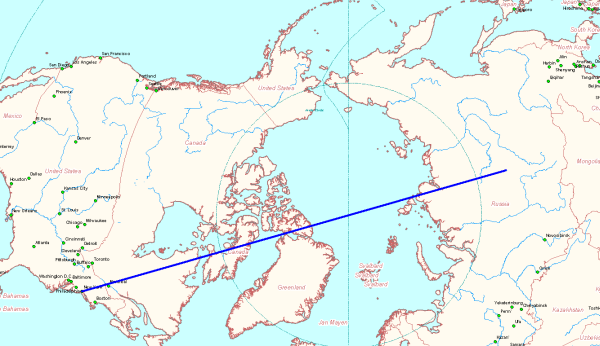#### Pole covering

A geodesic circle or ellipse can cover one or both poles. Extra segments are added to the representation of such figures to preserve closure when they're represented with lat-lon coordinates. The following two images show unprojected and projected views of an ellipse that covers the south pole. In the unprojected view, the extra segments connecting an extreme latitude line to the pole "line" were added to keep the figure closed. In the projected view, those segments show as a single short vertical line at the bottom of the ellipse.
In the first image, a geodesic ellipse is centered on Cape Town, is oriented north-south, and has a major axis 4000 miles in length. The wide base of the ellipse is the ellipse covering part of Antarctica and the South Pole.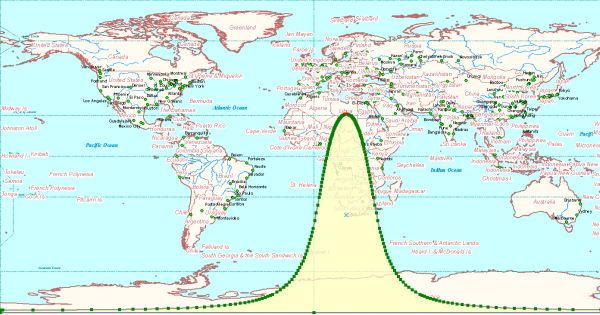The line segments along the -90 latitude at the bottom of this image are not removed from the projected ellipse. In the following image - which is a South Pole azimuthal equidistant projection - a line artificially extends from the south pole to the perimeter of the geodesic ellipse.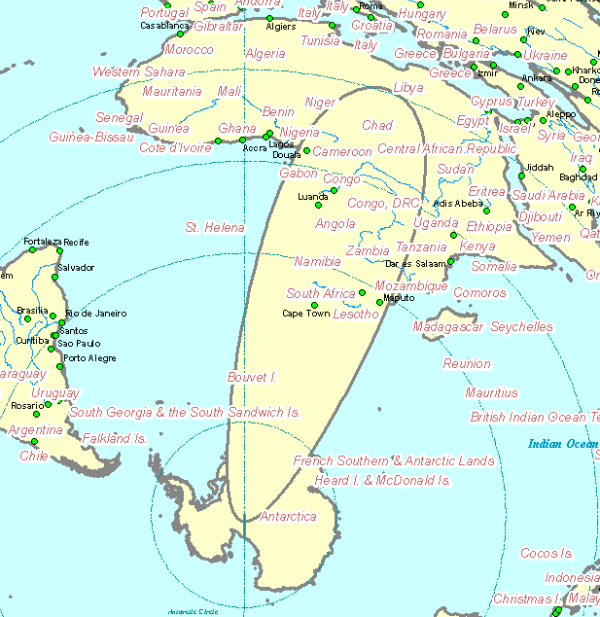A large ellipse or circle can cover both poles. A more extreme situation is when it covers a hemisphere. In this case, if it is being constructed as a polygon, an extra outer ring surrounding the entire GCS coordinate system (the GCS "horizon") is added to properly define the inside and outside of the polygon when it is stored with lat-lon coordinates. In the following image, the geodesic ellipse centered at Bangkok covers both poles. It has a major axis length of 9000 miles and is oriented north-south.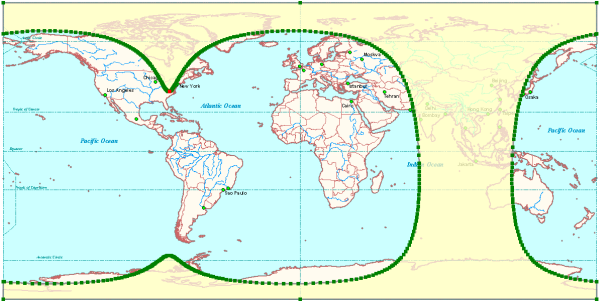#### Hemisphere covering

Another example of a geodesic ellipse that covers both poles is illustrated in the following image. In addition to covering both poles, this geodesic ellipse also completely covers the eastern hemisphere. This geodesic ellipse is represented as a two part polygon.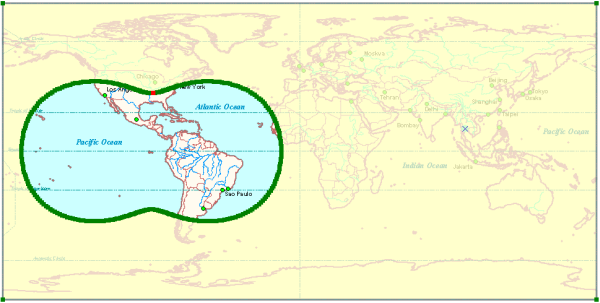Finally, an ellipse could form a "band" around the earth. This would be a case specific to an ellipse whose major axis is longer than the circumference of the earth. This would result in a polygon that is self-intersecting as shown in the following image. This last case is not currently dealt with.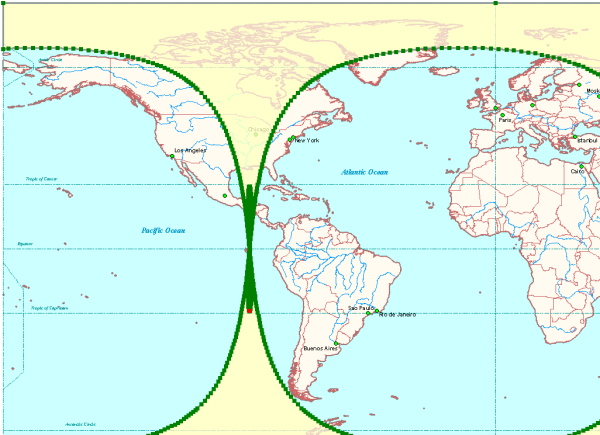### Densification methods

Geodetic curves and geodesic circles and ellipses are approximated by accurately placed vertices connected with planar straight lines. These lines typically do not follow the intended ground path of the geodetic curve. You control where the vertices are placed - and consequently, how much error is introduced in the representation of the ground path - by specifying a densification method and a densification parameter value.
Deviation densification lets you control the error directly. You specify the maximum distance allowed between the straight line and the geodetic curve. Vertices are generated and placed so that this maximum distance is never exceeded.
Angle densification lets you control the azimuth interval at which points are placed.
Length densification lets you place vertices along the curve at a specified length interval.
You can construct geodesic circles and ellipses using the deviation and angle densification methods, but not the length method. You can construct geodetic lines, and densify polycurves, using the length and deviation methods, but not the angle method.

#### Deviation

The deviation densification method lets you control the error introduced by the piecewise linear approximation to the geodetic curve. The following image demonstrates the deviation densification method with the geodetic curve represented by the curved black line and the maximum allowable error represented by the short, straight red line. The maximum allowable distance error (the deviation) is specified so that any individual straight line segment will not be perpendicularly separated from the true curve by more than the deviation distance.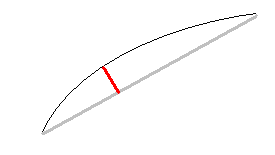In the following image, a geodesic line connects Bangkok and Tokyo. The distance between the true geodesic and the piecewise linear approximation is at most 0.5 mile. Additional vertices are used where the polyline curves the most. There are 28 vertices required to approximate the geodesic line between Tokyo and Bangkok with a maximum allowable offset of 0.5 mile.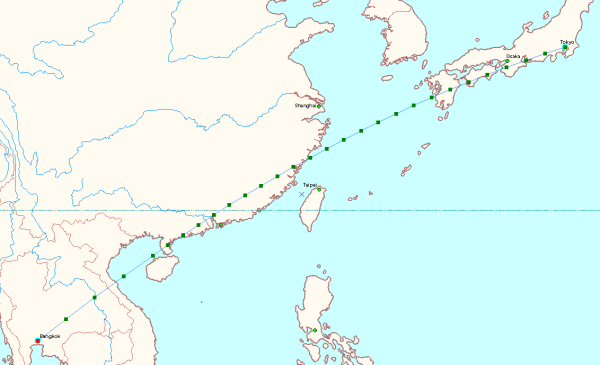#### Length

The following image is of a geodesic line approximation using length-based densification. This is the same line used in the deviation densification example only with points placed every 100 miles. In this case (length-based densification), 29 vertices are required, the maximum error is unknown, and the error with respect to the geodesic curve varies from line segment to line segment.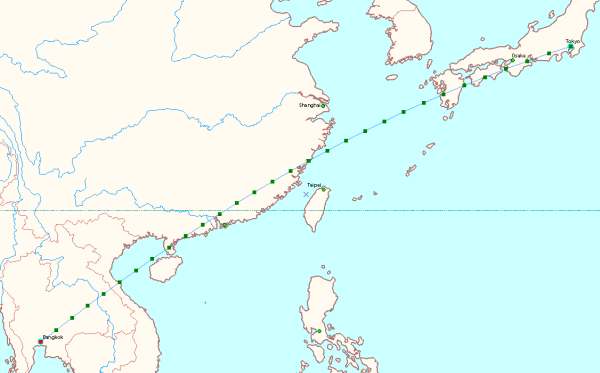#### Angle: circle vs. ellipse

When constructing geodesic circles and ellipses, the angle densification method can be used. With circles, the angle parameter is the difference in angle between the azimuths of two consecutive points. If you stand on the center point, look at a boundary point, then turn through the angle parameter value, you will be looking at the next point.
When constructing geodesic ellipses, the angle parameter cannot be interpreted this way. It is the angle on a unit reference circle (the inner circle in the following diagram). Points are plotted on a reference circle with the angle increment previously described, then scaled non-uniformly onto the boundary of the ellipse (as described in the About geodetic curves section at the beginning of this topic). The final azimuth to the ellipse point is different than the azimuth used to create the point on the reference circle.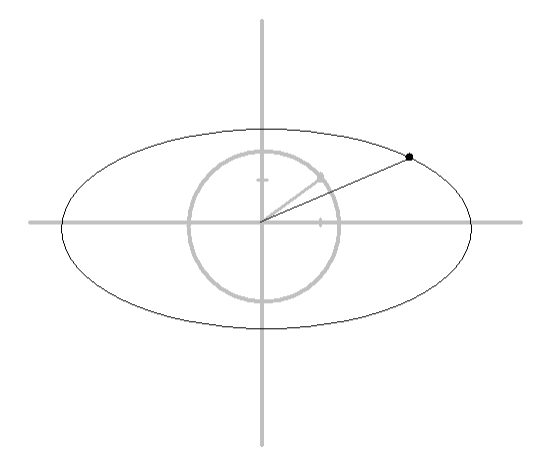Use the deviation densification method for circles and ellipses. It is the most efficient and will use the least number of points necessary to maintain the specified level of ground accuracy.
In the following image, a geodesic circle is created with the angle densification method. The difference in azimuths between each successive pair of boundary points is a constant.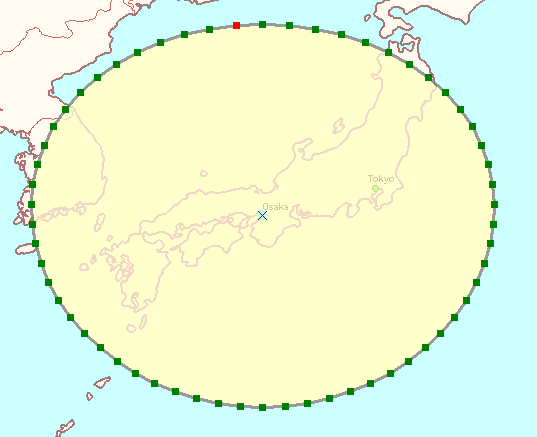In the following image, a geodesic ellipse is created with the angle densification method. The difference in azimuths between each successive pair of boundary points is not constant.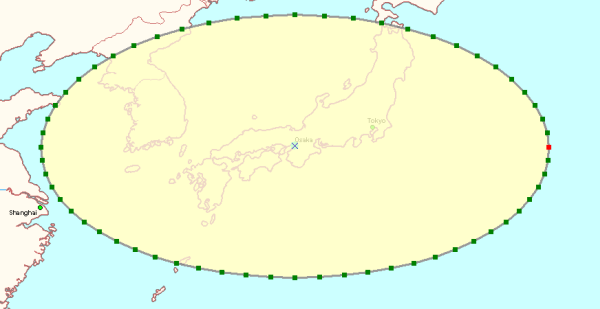### Code sample

The following code sample shows how to create a geodesic ellipse:
[VB.NET]
``````Private Shared Function CreateGeodeticEllipse() As IPolygon
Dim polygon As IPolygon
polygon=New Polygon
Dim polygonCG As IConstructGeodetic
polygonCG=polygon
Dim t As Type=Type.GetTypeFromProgID("esriGeometry.SpatialReferenceEnvironment")
Dim obj As System.Object=Activator.CreateInstance(t)
Dim spatialReferenceFactory As ESRI.ArcGIS.Geometry.ISpatialReferenceFactory3=obj
Dim spatialReference As ISpatialReference
spatialReference=spatialReferenceFactory.CreateSpatialReference(esriSRGeoCSType.esriSRGeoCS_WGS1984)
Dim linearUnitMiles As ILinearUnit
linearUnitMiles=spatialReferenceFactory.CreateUnit(esriSRUnitType.esriSRUnit_SurveyMile)
Dim centerPoint As Point
centerPoint=New Point
centerPoint.X=45D
centerPoint.Y=45D
centerPoint.SpatialReference=spatialReference
polygonCG.ConstructGeodesicEllipse(centerPoint, linearUnitMiles, 2000D, 1000D, 90D, esriCurveDensifyMethod.esriCurveDensifyByDeviation, 1D)
Return polygon
End Function```
```
[C#]
``````public static IPolygon CreateGeodeticEllipse()
{
IPolygon polygon=new PolygonClass();
IConstructGeodetic polygonCG=(IConstructGeodetic)polygon;
Type factoryType=Type.GetTypeFromProgID(
"esriGeometry.SpatialReferenceEnvironment");
System.Object obj=Activator.CreateInstance(factoryType);
ISpatialReferenceFactory3 spatialReferenceFactory=obj as
ISpatialReferenceFactory3;
ISpatialReference spatialReference =
spatialReferenceFactory.CreateSpatialReference((int)
esriSRGeoCSType.esriSRGeoCS_WGS1984);
ILinearUnit linearUnitMiles=(ILinearUnit)spatialReferenceFactory.CreateUnit(
(int)esriSRUnitType.esriSRUnit_SurveyMile);
IPoint centerPoint=new PointClass();
centerPoint.X=45d;
centerPoint.Y=45d;
centerPoint.SpatialReference=spatialReference;
polygonCG.ConstructGeodesicEllipse(centerPoint, linearUnitMiles, 2000d,
//Semi-major axis length.
1000d,  //Semi-minor axis length.
90d,  //Semi-major axis azimuth.
esriCurveDensifyMethod.esriCurveDensifyByDeviation, 1d); //Densify parameter.
return polygon;
}```
```
The following image shows the geodesic ellipse created by this code sample: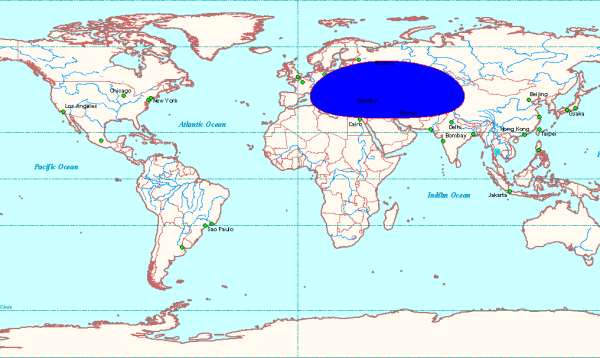### Enumerations

The well-known IDs for the various linear and angular units supported by ArcGIS are located in the esriSRUnitType and esriSRUnit2Type enumerations.

To use the code in this topic, reference the following assemblies in your Visual Studio project. In the code files, you will need using (C#) or Imports (VB .NET) directives for the corresponding namespaces (given in parenthesis below if different from the assembly name):

Development licensing Deployment licensing
Engine Developer Kit Engine